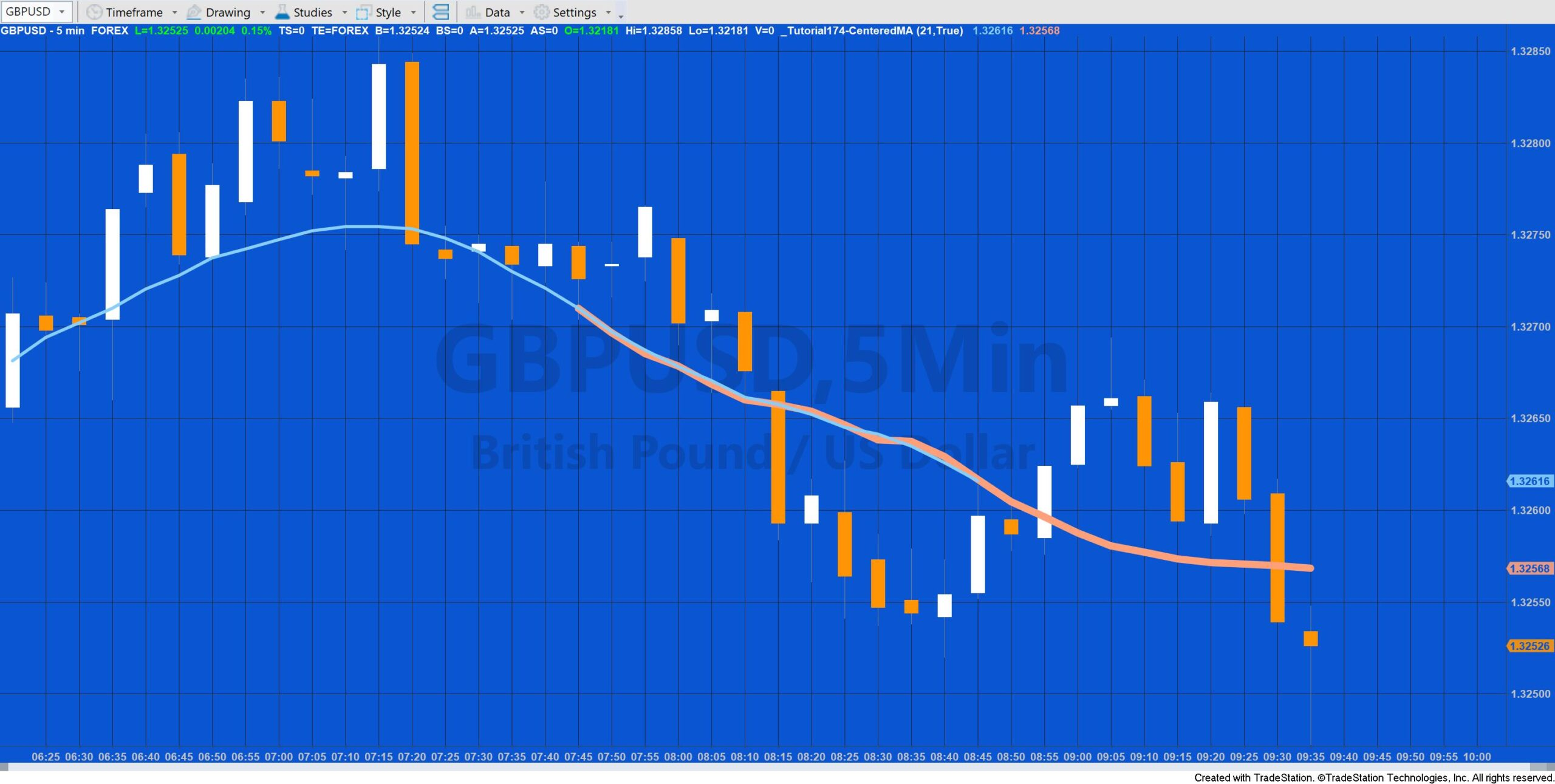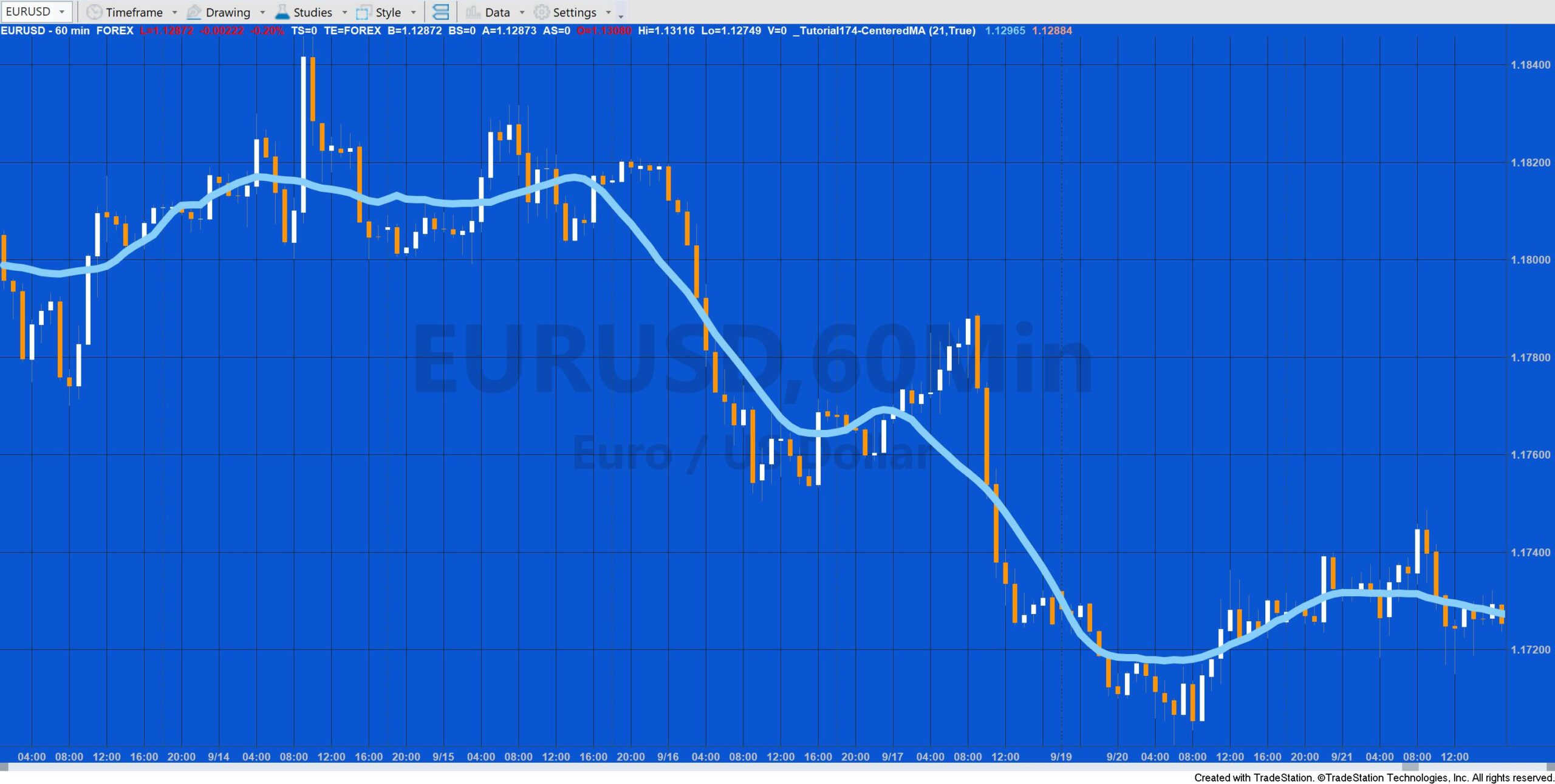# Tutorial 174 | Markplex Centered Moving Average

Tutorial 174 demonstrates how to write Markplex’s version of the Centered Moving Average (CMA) using TradeStation EasyLanguage. A centered moving average is placed at the center of the range rather than the end of it. This positions the moving average values at their central positions in time and is easy to accomplish by using EasyLanguage’s displace capability.

A disadvantage of the CMA is that because the values are displaced there are a number of bars at the end of the chart where there are not a sufficient number of future bars to calculate the average, by definition.

### Estimating the CMA for ‘missing’ bars

This tutorial demonstrates one approach to estimating the average for the last bars by replacing “missing” bars with the Close of the last bar and then re-calculating and re-plotting the last few bars whenever the close is updated.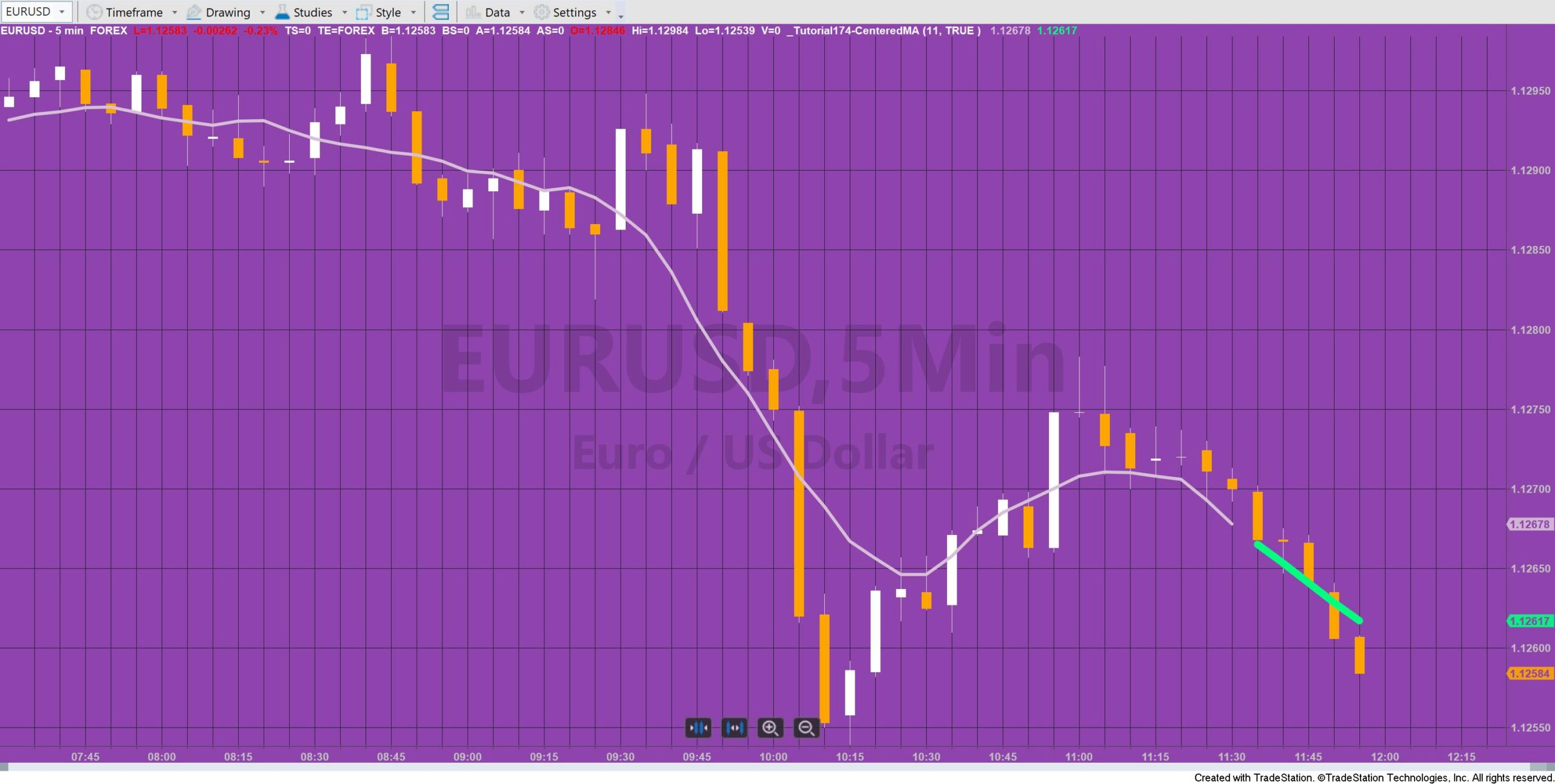Here, the estimated CMA is plotted in green

Estimates are made for the CMA for last few bars on the chart. These bars are plotted in a different color, by default. In the example below the displaced plot is plotted in light magenta and the estimated bars are plotted in green.  The earliest bar of the estimate remains on the chart after a new bar develops (hence the green plot is longer than 5 bars – the length in this example was 11.)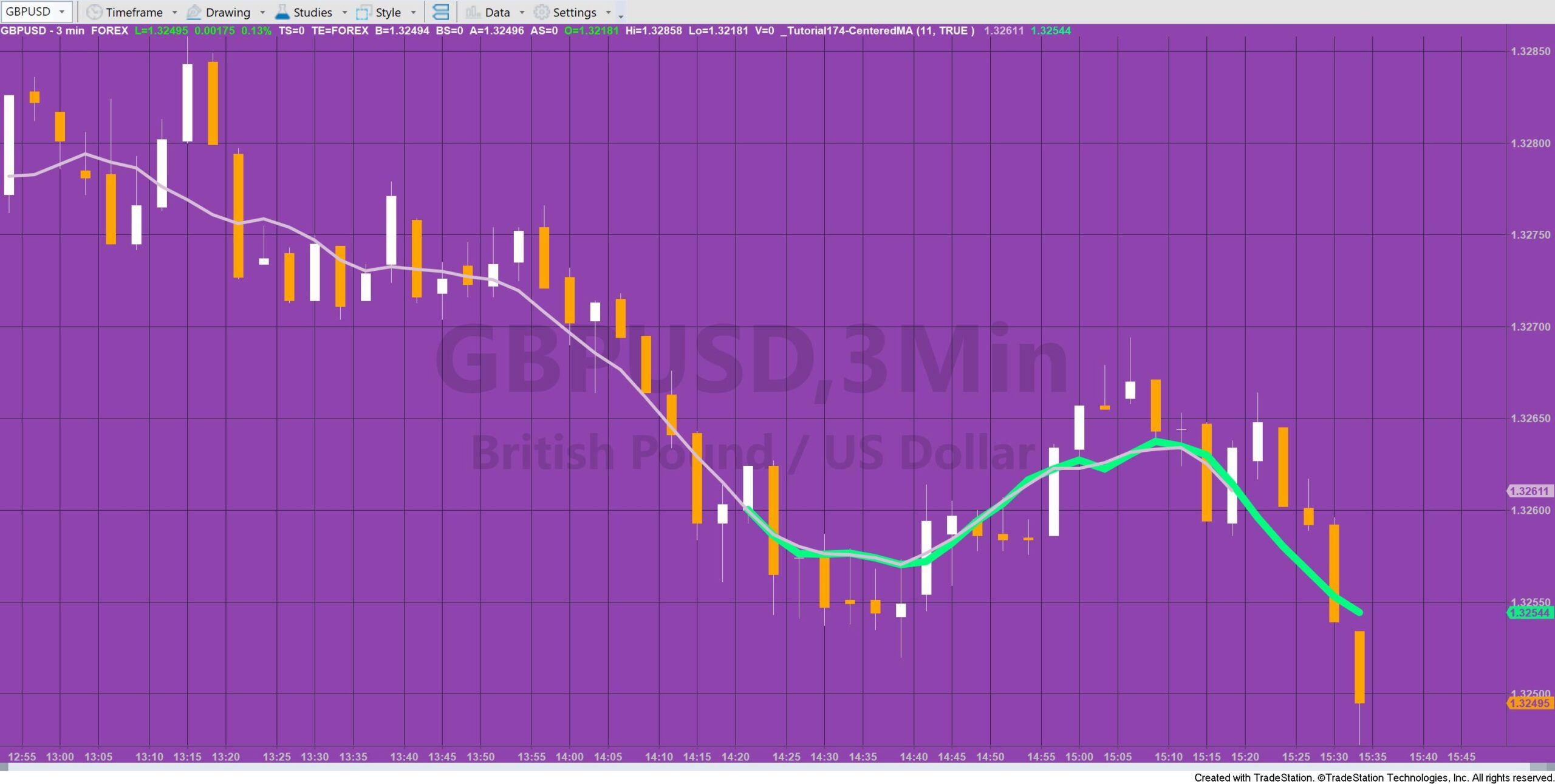Tutorial 173 can be used with TradeStation OR with MultiCharts.

### Odd or even number of bars?

When Length is an even number (i.e. there is a no bar that is in the center of the length) the plot is plotted one bar later. For example, if Length is equal to 10 and it is the last bar on the chart, the plot for the center moving average is made 4 bars ago and 4 estimated values are calculated and plotted.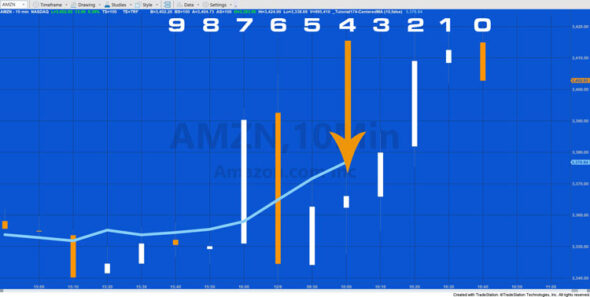In the case when the length is an odd number then the middle bar has an equal number of bars on either side. For example, in this example, Length is set to 11. The middle bar is number 5 (remember that the bars are counted from zero.: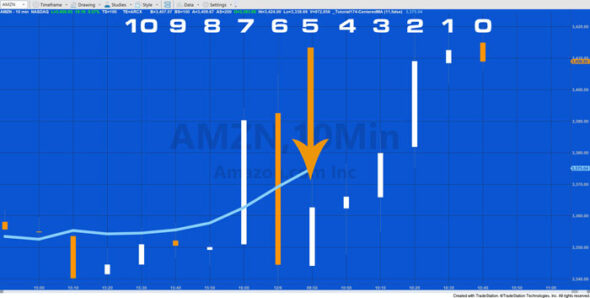i.e. 5 bars on either side of the center bar.

Estimates are made for the CMA for last few bars on the chart. These bars are plotted in a different color, by default. In the example below the displaced plot is plotted in light magenta and the estimated bars are plotted in green.  The earliest bar of the estimate remains on the chart after a new bar develops (hence the green plot is longer than 5 bars – the length in this example was 11.)

## Tutorial 174 Overview Video

See a video walk through of tutorial 174.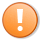This content is for members only.